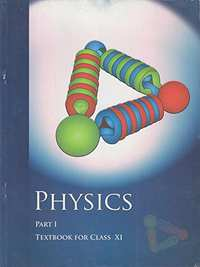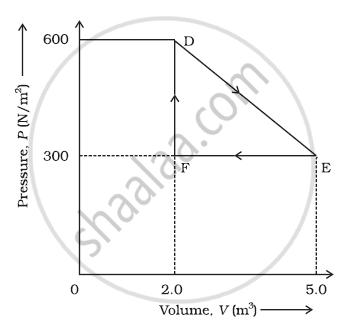CBSE (Science) Class 11CBSE
Share

NCERT solutions for Class 11 Physics chapter 12 - Thermodynamics

Physics Textbook for Class 11

NCERT Physics Class 11Chapter 12: Thermodynamics

Chapter 12: Thermodynamics solutions [Pages 0 - 317]

In changing the state of a gas adiabatically from an equilibrium state to another equilibrium state B, an amount of work equal to 22.3 J is done on the system. If the gas is taken from state to via a process in which the net heat absorbed by the system is 9.35 cal, how much is the net work done by the system in the latter case? (Take 1 cal = 4.19 J)

A geyser heats water flowing at the rate of 3.0 litres per minute from 27 °C to 77 °C. If the geyser operates on a gas burner, what is the rate of consumption of the fuel if its heat of combustion is 4.0 × 104 J/g?

What amount of heat must be supplied to 2.0 x 10-2 kg of nitrogen (at room temperature) to raise its temperature by 45 °C at constant pressure? (Molecular mass of N2 = 28; R = 8.3 J mol-1 K-1.)

Explain why Two bodies at different temperatures T1 and T2, if brought in thermal contact, do not necessarily settle to the mean temperature (T1 + T2)/2.

The coolant in a chemical or a nuclear plant (i.e., the liquid used to prevent the different parts of a plant from getting too hot) should have high specific heat.

Explain why Air pressure in a car tyre increases during driving.

Explain why The climate of a harbour town is more temperate than that of a town in a desert at the same latitude.

A cylinder with a movable piston contains 3 moles of hydrogen at standard temperature and pressure. The walls of the cylinder are made of a heat insulator, and the piston is insulated by having a pile of sand on it. By what factor does the pressure of the gas increase if the gas is compressed to half its original volume?

In changing the state of a gas adiabatically from an equilibrium state to another equilibrium state B, an amount of work equal to 22.3 J is done on the system. If the gas is taken from state to via a process in which the net heat absorbed by the system is 9.35 cal, how much is the net work done by the system in the latter case? (Take 1 cal = 4.19 J)

Two cylinders and of equal capacity are connected to each other via a stopcock. contains a gas at standard temperature and pressure. is completely evacuated. The entire system is thermally insulated. The stopcock is suddenly opened. Answer the following:

(a) What is the final pressure of the gas in and B?

(b) What is the change in internal energy of the gas?

(c) What is the change in the temperature of the gas?

(d) Do the intermediate states of the system (before settling to the final equilibrium state) lie on its P-V-T surface?

A steam engine delivers 5.4×10J of work per minute and services 3.6 × 10J of heat per minute from its boiler. What is the efficiency of the engine? How much heat is wasted per minute?

An electric heater supplies heat to a system at a rate of 100W. If the system performs work at a rate of 75 Joules per second. At what rate is the internal energy increasing?

A thermodynamic system is taken from an original state to an intermediate state by the linear process shown in FigureIts volume is then reduced to the original value from E to F by an isobaric process. Calculate the total work done by the gas from D to E to F

A refrigerator is to maintain eatables kept inside at 9°C. If the room temperature is 36° C, calculate the coefficient of performance.

Chapter 12: Thermodynamics

NCERT Physics Class 11NCERT solutions for Class 11 Physics chapter 12 - Thermodynamics

NCERT solutions for Class 11 Physics chapter 12 (Thermodynamics) include all questions with solution and detail explanation. This will clear students doubts about any question and improve application skills while preparing for board exams. The detailed, step-by-step solutions will help you understand the concepts better and clear your confusions, if any. Shaalaa.com has the CBSE Physics Textbook for Class 11 solutions in a manner that help students grasp basic concepts better and faster.

Further, we at Shaalaa.com are providing such solutions so that students can prepare for written exams. NCERT textbook solutions can be a core help for self-study and acts as a perfect self-help guidance for students.

Concepts covered in Class 11 Physics chapter 12 Thermodynamics are Isothermal Processes, Adiabatic Processes, Thermal Equilibrium, Zeroth Law of Thermodynamics, Heat, Internal Energy and Work, First Law of Thermodynamics, Specific Heat Capacity, Thermodynamic State Variables and Equation of State, Thermodynamic Processes, Heat Engines, Refrigerators and Heat Pumps, Second Law of Thermodynamics, Reversible and Irreversible Processes, Carnot Engine.

Using NCERT Class 11 solutions Thermodynamics exercise by students are an easy way to prepare for the exams, as they involve solutions arranged chapter-wise also page wise. The questions involved in NCERT Solutions are important questions that can be asked in the final exam. Maximum students of CBSE Class 11 prefer NCERT Textbook Solutions to score more in exam.

Get the free view of chapter 12 Thermodynamics Class 11 extra questions for Physics and can use Shaalaa.com to keep it handy for your exam preparation

S